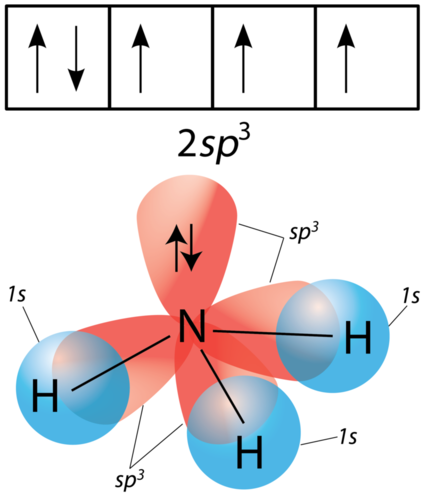Prediction:Hybridizationsource : ck 12.org

## Prediction of Hybridization Geometry (shape) of Covalent Molecules :

There are two method :

## First Method:

H = number of sigma bond + number of lone pair of  electrons at that atom

If value of  ‘H’ is two then sp , three then sp2, four then sp3.

Example: 1 )  BeCl2

Number of sigma bond at Be atom = 2

Number of lone pair of electrons at Be atom = 0

H = number of sigma bond + number of lone pair electrons at that atom

=2+0 =2 (sp hybridization, angle 180 degree and  geometry of molecule is linear)

Example: 2)  CCl4

Number of sigma bond = 4

Number of lone pair of electrons at C- atom = 0

H = number of sigma bond + number of lone pair of  electrons at that atom

=4+0 =4 (sp3 hybridization, angle 109o28 and  shape of molecule is Tetra hedral)

Example 3:  NH3

number of sigma bond = 3

number of lone pair of electrons at N-atom = 1

H = number of sigma bond + number of lone pair electrons at that atom

=3+1 =4 ( sp3 hybridization , angle 109 o28 and geometry is Tetra hedral)

Example: 4)  H2O

number of sigma bond = 2

number of lone pair of electrons at O-atom  = 2

H = number of sigma bond + number of lone pair electrons at that atom

=2+2 =4 (sp3 hybridization, angle 109 o28 and  shape is Tetra hedral)

## Alternate Method :

H= [ (V+M – C  +A)]/2

H = number of orbitals involved in hybridization

if  H = 2 then sp , if H = 3 then sp2, if H = 4 then sp3, if H = 5 then sp3d, if H = 6 then sp3d2

V = Number of electrons in valence shell of central atom.

M = Number of mono-valent atoms

C = Charge on cation

A = Charge on anion

Example:1)  NH4+

H= [ (V+M – C  +A)]/2

V= 5 ; M =4 ; C=1; A =0

H =  (5 +4 -1 +0)/2

=4 (sp3 hybridization)

Example: 2)  PO43-

H= [ (V+M – C  +A)]/2

V= 5 ; M =0 (because oxygen is divalent ) ; C=0; A =3

H =  (5 +0-0 +3)/2

=4 (sp3 hybridization)

Example: 3)  SO2-

H= [ (V+M – C  +A)]/2

V= 6 ; M =0 (because oxygen is divalent ) ; C=0; A =2

H =  (6 +0-0 +2)/2

=4 (sp3 hybridization)

Example: 4)  SF6

H= [ (V+M – C  +A)]/2

V= 6 ; M =6 ; C=0; A =0

H =  (6 +6 – 0 +0)/2

=6 (sp3d2 hybridization)

Example: 5) PCl5

H= [ (V+M – C  +A)]/2

V= 5 ; M =5 ; C=0; A =0

H =  (5 +5 -0 +0)/2

=5 (sp3d hybridization)

V= 6 ; M =0 ; C=0; A =2